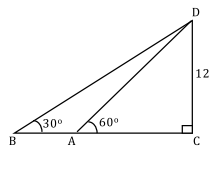Your Insider Guide to the GRE

[not sponsored or endorsed by the ETS]

# Free GRE Test Questions

Question 1 of 1
ID: GRE-MC-2
Section: Quantitative Reasoning - Multiple Choice with one answer
Topics: Geometry; Triangles
Difficulty level: Medium

(Practice Mode: Single selected Question » Back to Overview)

In the diagram shown above, if CD = 12, what is the length of AB?A$3\sqrt{3}$
B$4\sqrt{3}$
C$8\sqrt{3}$
D$12\sqrt{3}$
E$16\sqrt{3}$